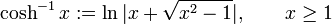# Inverse hyperbolic cosine function

The inverse hyperbolic cosine function, denoted$\cosh^{-1}$, is a function with domain the set$[1,\infty)$, defined in either of the following equivalent ways:
1. It is the inverse function to the restriction of the hyperbolic cosine function$\cosh$ to the subset$[0,\infty)$.$\cosh^{-1} x := \ln|x + \sqrt{x^2 - 1}|, \qquad x \ge 1$